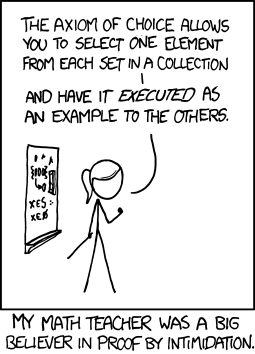Programmer's Guide To Theory - The Algorithm of Choice
Written by Mike James
Tuesday, 15 August 2023
Article Index
Programmer's Guide To Theory - The Algorithm of Choice
To Infinity And...
Non-Constructive

The axiom of choice is the most esoteric math concept you are likely to encounter. You might think it has no relevance to computing, but you would be wrong.

## A Programmers Guide To Theory

#### Now available as a paperback and ebook from Amazon.#### Contents

1. What Is Computer Science?
Part I What Is Computable?
2. What Is Computation?
3. The Halting Problem
4. Finite State Machines
Extract 1: Finite State Machines
5. Practical Grammar
6. Numbers, Infinity and Computation
Extract 1: Numbers
Extract 2: Aleph Zero The First Transfinite
Extract 3: In Search Of Aleph-One
Extract 4: Transcendental Numbers
7. Kolmogorov Complexity and Randomness
Extract 1:Kolmogorov Complexity
8. The Algorithm of Choice
9. Gödel’s Incompleteness Theorem
10. Lambda Calculus ***NEW!
Part II Bits, Codes and Logic
11. Information Theory
12. Coding Theory – Splitting the Bit
13. Error Correction
14. Boolean Logic
Part III Computational Complexity
15. How Hard Can It Be?
Extract 1: Where Do The Big Os Come From
16. Recursion
Extract 1: What Is Recursion
Extract 2: Why Recursion
17. NP Versus P Algorithms
Extract 1: NP & Co-NP
Extract 2: NP Complete

<ASIN:1871962439>

<ASIN:1871962587>

After looking at some advanced math concerning infinity, you can start to appreciate that algorithms, or programs, have a lot to do with math. The fact that the number of programs is smaller than the number of numbers gives rise to many mathematical phenomena. One particularly exotic mathematical idea, known as the axiom of choice, isn’t often discussed in computer science circles and yet it probably deserves to be better known. However, this said, if you want to stay close to a standard account of computer science, you are free to skip this chapter.

Before we get started, I need to say that this isn't a rigorous mathematical exposition of the axiom of choice. What it is attempting to do is to give the idea of the "problem" to a non-mathematician, i.e. an average programmer. I also need to add that while there is nothing much about the axiom of choice that a practical programmer needs to know to actually get on with programming it, it does have connections with computer science and computability. The axiom of choice may not be a particularly practical sort of mathematical concern, but it is fascinating and it is controversial.

## Zermelo and Set Theory

The axiom of choice was introduced by Ernst Zermelo in 1904 to prove what seems a very reasonable theorem.Ernst Zermelo
1871-1953

In set theory you often find that theorems that seem to state the obvious actually turn out to be very difficult to prove. In this case the idea that needed a proof was the "obvious" fact that every set can be well ordered. That is, there is an order relation that can be applied to the elements so that every subset has a least element under the order. This is Zermelo's well-ordering theorem. To prove that this is the case Zermelo had to invent the axiom of choice. It now forms one of the axioms of Zermelo-Fraenkel set theory which is, roughly speaking, the theory that most would recognize as standard set theory. There are a lot of mathematical results that depend on the axiom of choice, but notice it is an axiom and not a deduction. That is, despite attempts to prove it from simpler axioms, no proof has ever been produced.  Mathematicians generally distinguish between Zermelo-Fraenkel set theory without the axiom of choice and a bigger theory where more things can be proved with the axiom of choice.More cartoon fun at xkcd a webcomic of romance,sarcasm, math, and language

## The Axiom of Choice

So exactly what is the axiom of choice - it turns out to be surprisingly simple and if you read the cartoon caption you will find that it tells you nearly everything you need to know:

"The axiom of choice allow you to select one element from each set in a collection"

- yes, it really is that simple.

A countable collection of sets is just an enumeration of sets Si for a range of values of i. The axiom of choice says that for each and every i you can pick an element from the set Si. It is so obvious that it hardly seems worth stating as an axiom - but it has a hidden depth.

Another way of formulating the axiom of choice is to say that for any collection of sets there exists a choice function f which selects one element from each set, i.e. f(Si) is an element of Si.

Notice that if you have a collection of sets that comes with a natural choice function then you don't need the axiom of choice. The fact you have an explicit choice function means that you have a mechanism for picking one element for each set. The axiom of choice is a sort of reassurance that even if you don't have an explicit choice function one exists - you just don't know what it is.

Another way to look at this is that the axiom of choice says that a collection of sets for which there is no choice function doesn't exist.

Last Updated ( Tuesday, 15 August 2023 )